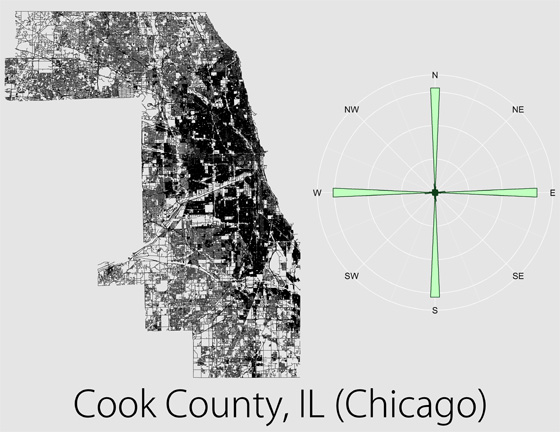## The Chicago grid is almost perfectly aligned with true North.

Rate this fact:All calculations were made in a sinusoidal map projection using the central longitude of the area of interest. I then graphed the angles on rose diagrams (wind roses) using bins of 5 degrees to show relative distributions for each area. The plots were scaled such that the maximum bar height was the same on each rose. To ensure rotational symmetry in the plots, each azimuth was counted twice: once using the original value and once using the opposite direction (e.g., 35 degrees and 215 degrees). As such, all streets, regardless of one-way or two-way traffic, were considered to be pointing in both directions.